# Assignments

Below a number of assignments about lines, their slopes, when they are in parallel, how you can calculate the formula of a line through a given point and slope or through two given points.

1. Draw the line: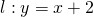and calculate the slope and the exact intersection point of the line with the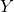-as.

Solution

2. Draw the line: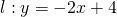and calculate the slope and the exact intersection point of the line with the-as.

Solution

3. Draw the line: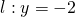and calculate the slope and the exact intersection point of the line with the-as.

Solution

4. Draw the line of the following linear functions: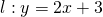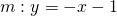and calculate their intersection point.

Solution

5. Draw the lines of the following linear functions: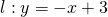and calculate their intersection point.

Solution

6. The linethrough the point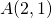with slope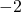intersects the line: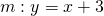in point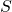.
Calculate the coordinates of.

Solution

7. The linegoes through the pointsand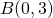and intersects the line: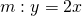in point.
Calculate the coordinates of.

Solution

8. The linegoes through the points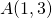and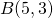and intersects the line: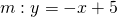in the point.
Calculate the coordinates of.

Solution

9. Given the following two linear equations with two unknown variablesen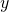: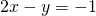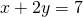1. The equations represent lines. Calculate their slopes.
2. How can you calculate the coordinates of their intersection point?

Solution

10. The linegoes through the points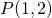and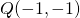. For which value of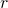is the line: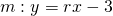parallel to?

Solution

0